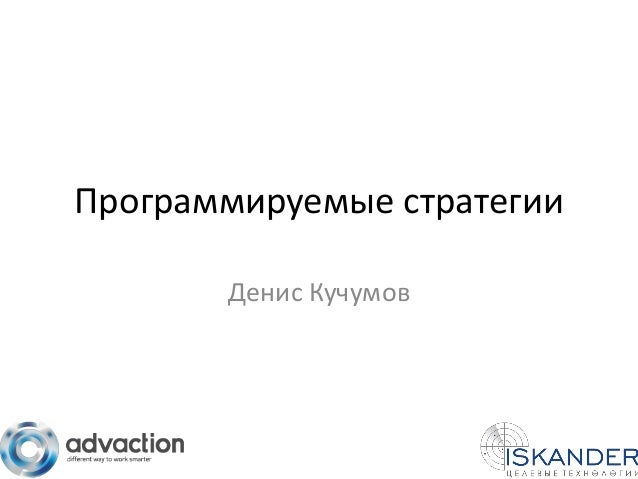Successfully reported this slideshow.Upcoming SlideShare
×

# РИФ 2016, Программируемые стратегии в таргетированной рекламе.

Денис Кучумов
Iskander

• Full Name
Comment goes here.

Are you sure you want to Yes No• Be the first to like this

### РИФ 2016, Программируемые стратегии в таргетированной рекламе.

1. 1. Программируемые стратегии Денис Кучумов
2. 2. Типы целей 1. Оптимизация по доходу. 2. Оптимизация по времени. 3. Это модно (люблю смотреть на цифры).
3. 3. Этап 1. Безбюджетный арбитраж http://calculate.iskander.su/
4. 4. Этап 1. Безбюджетный арбитраж http://calculate.iskander.su/
5. 5. Этап 1. Безбюджетный арбитраж http://calculate.iskander.su/
6. 6. Этап 2. Запуск и проведение кампаний. http://calculate.iskander.su/
7. 7. Этап 2. Запуск и проведение кампаний. http://calculate.iskander.su/
8. 8. Этап 2. Запуск и проведение кампаний.
9. 9. Этап 3. Стратегия. Тестирования креатива Активировать стратегию, если показы > 1000. Остановить объявление, если ctr < 1.
10. 10. Стратегия. Прибыльные баннеры Активировать стратегию, если потрачено > 500 рублей. Остановить объявление, если roi < 50%, запустить, если roi >= 50. http://calculate.iskander.su/
11. 11. Когда нужна стратегия? 1. Есть данные для работы. 2. Понятна конечная цель. 3. Есть алгоритм действий. http://calculate.iskander.su/
12. 12. Спасибо за внимание Денис Кучумов +7 (495) 795-77-39 expert@advaction.ru http://www.iskander.su https://www.youtube.com/cpanettv